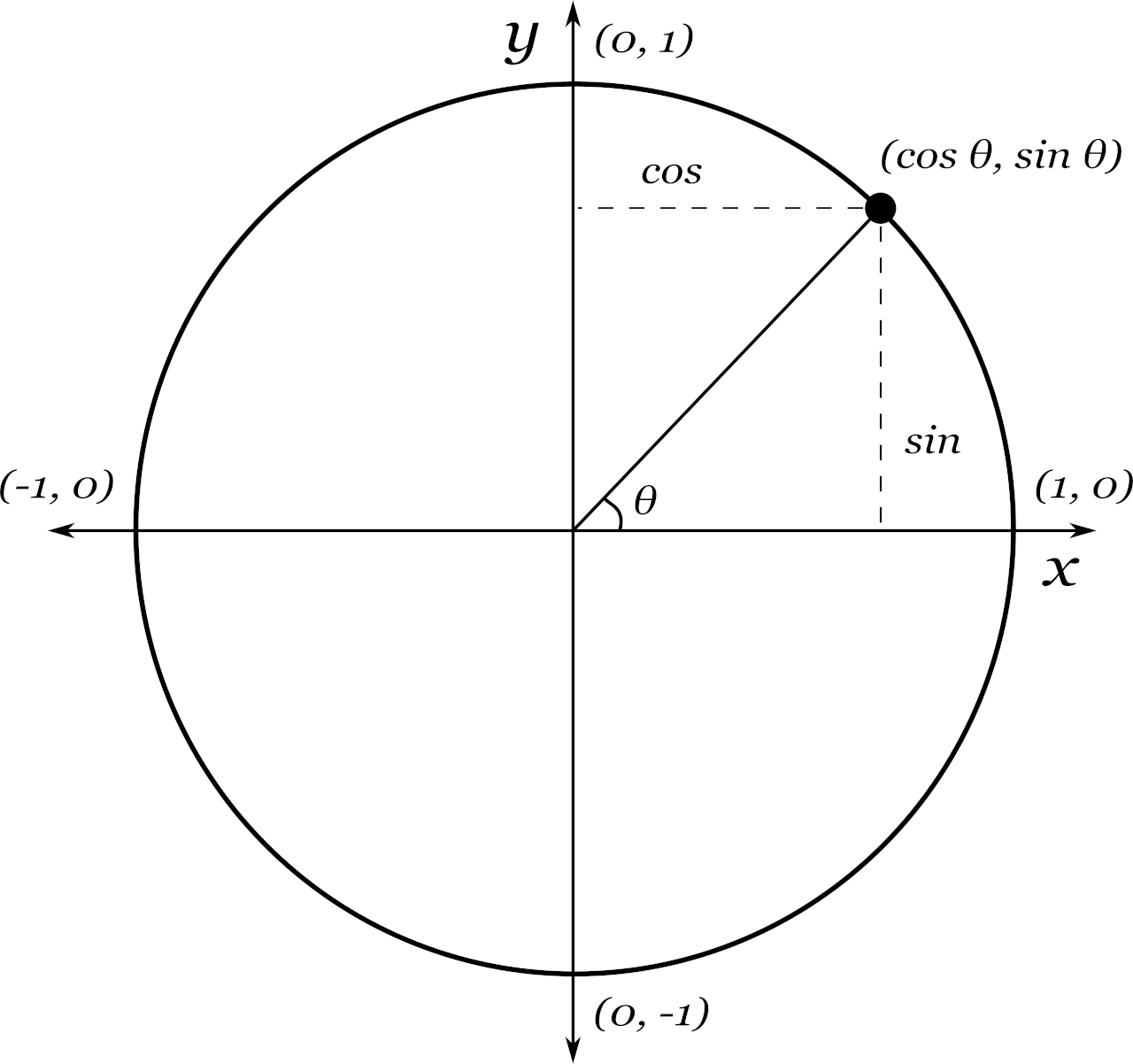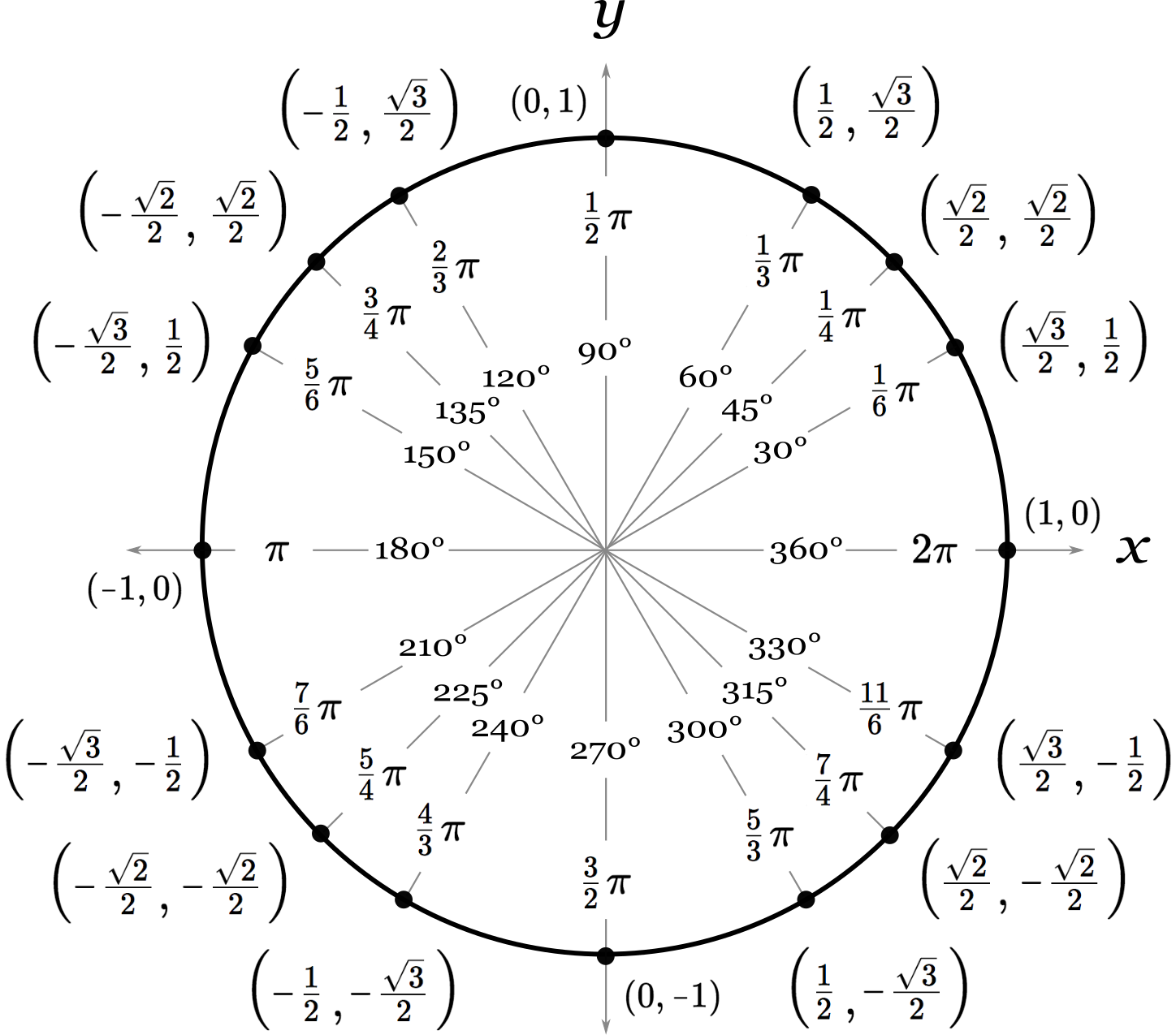# Unit Circle Calculator

Calculate the coordinates for a point on the unit circle given the central angle in radians or degrees. You’ll also get the sine, cosine, and tangent in the results.## The Unit Circle

The unit circle is a circle with a radius equal to 1 and is centered at the origin. It is sometimes also referred to as the trig circle because it’s used t calculate the cosine, sine, and tangent of any angle within the circle.The unit circle defines how to solve the parts of a right triangle formed when extending a line for a known angle within the circle.

Since the radius of the unit circle is 1, the right triangle’s hypotenuse is equal to 1.

The edge of the triangle (leg a) is equal to the sine of the angle, while the base of the triangle (leg b) is equal to the cosine.

## How to Find Coordinates on the Unit Circle

The point, or coordinate, where the radius at the defined angle intersects the circle can also be calculated using trigonometric functions.

As noted above, the edge of the right triangle formed is equal to the sine of the angle θ; this becomes the y-coordinate. The base of the triangle is equal to the cosine of the angle, which becomes the x-coordinate.

Thus, the coordinate where the radius intersects the circle is:

(cos θ, sin θ)

## Unit Circle Chart with Radians and Degrees

The unit circle chart shows the angles used in the 30-60-90 and 45-45-90 special right triangles, and the coordinates where the radius intersects the edge of the unit circle.The chart shows the angles in radians and degrees, and shows each coordinate solved using the special right triangle created using the unit circle.

### Unit Circle Chart Table

Table showing the angles and coordinates in the unit circle chart.
Angle (degrees) Angle (radians) Unit Circle Coordinates
30° π6 (√32, 12)
45° π4 (√22, √22)
60° π3 (12, √32)
90° π2 (0, 1)
120° 3 (-12, √32)
135° 4 (-√22, √22)
150° 6 (-√32, 12)
180° π (-1, 0)
210° 6 (-√32, –12)
225° 4 (-√22, –√22)
240° 3 (-12, –√32)
270° 2 (0, -1)
300° 3 (12, –√32)
315° 4 (√22, –√22)
330° 11π6 (√32, –12)
360° (1, 0)

## Tricks to Remember the Unit Circle

The unit circle might intimidate you, but remembering it might be easier than it might seem at first glance. There are a few tricks you can use to memorize the unit circle.

Try these tricks to memorize the unit circle without needing to remember every coordinate.

The following table shows degree to radian conversions for the angles in the unit circle.

Table showing degree to radian conversions for the unit circle.
30° π6
45° π4
60° π3
90° π2
120° 3
135° 4
150° 6
180° π
210° 6
225° 4
240° 3
270° 2
300° 3
315° 4
330° 11π6
360°

### Trig Functions for Common Angles

The following table shows common angles and the resulting values using the trig functions for the top right quadrant of the unit circle. You’ll notice these coordinates and their negative values repeated for the entire unit circle.

Table showing angles and resulting trig function values.
Angle (θ) Trig Functions
degrees radians sin θ cos θ
30° π6 12 √32
45° π4 √22 √22
60° π3 √32 12